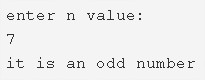# C Program To Check Whether A Number Is Even Or Odd | C Programs

C program to check whether a number is even or odd – In this article, we will discuss the method to check whether a number is even or odd in C programming.

Suitable examples and sample programs have also been added so that you can understand the whole thing very clearly. The compiler has also been added with which you can execute it yourself.

The method used in this piece is as follows:

• Using Standard Method

As well all know, an even number is a number which is divisible by 2 and does not leave behind a remainder.

However, an odd number is a number is a number which isn’t divisible by 2 and leaves behind a remainder.As we can see in the image uploaded above, you need to enter a value which you wanna check whether it is even or odd.

The number which is mentioned is 7.

It is evident that 7 is an odd number.

Thus, the same to do in C programming is as follows:

## Using Standard Method

1)Read the entered number and store in the variable n using scanf function.

2)If n is divisible by 2 then print “it is an even number”, otherwise print “it is an odd number”.

Output:
x

## C Program To Count The Total Number Of Notes In A Amount | C Programs

C program to count the total number of notes in a given amount – In ...